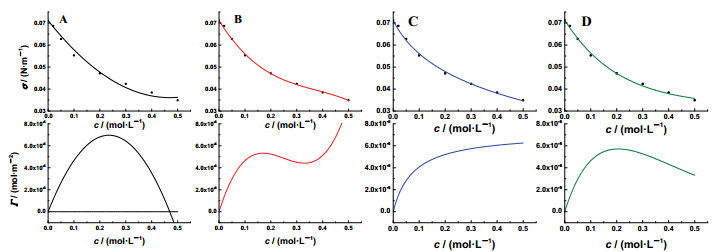## Revisiting the Solution Adsorption Experiment,, ,

 基金资助: 中国高等教育学会高等教育科学研究"十三五"规划课题.  16SY004

 Fund supported: 中国高等教育学会高等教育科学研究"十三五"规划课题.  16SY004Abstract

In the solution adsorption experiment, different adsorption mode has been observed for aqueous ethanol and aqueous n-butanol, respectively. However, it is difficult to make an adjustment by the profiles of σ-c relationship. Choosing the experimental conditions improperly will give wrong results. Since there is no consistency between the Gibbs adsorption isotherm and Langmuir adsorption isotherm, the experimental data should not be fitted by using any algebraic equations or physical models. The data fitting for this experiment should be carried out according to the following steps:fitting the σ-lnc curve manually, making it monotonic and smooth; retrieving more data from this fitting curve, and re-plotting the σ-lnc curve; calculating the first derivatives at these data points (i.e., (∂σ/lnc)T); calculating surface adsorptions by Gibbs adsorption isotherm, Γ=-(∂σ/lnc)T/RT. As for the calculation of the cross-section area for the solute molecule, the effect of concentration of bulk solution (i.e., c) on the surface molecular density should be taken into account.

XU Xinhua, WU Meifen, WANG Xiaogang. Revisiting the Solution Adsorption Experiment. University Chemistry[J], 2018, 33(10): 91-96 doi:10.3866/PKU.DXHX201803038

## 1 正丁醇和乙醇水溶液的表面吸附

$\mathit{\Gamma} = - \frac{1}{{RT}}{\left( {\frac{{\partial \sigma }}{{\partial \ln \alpha }}} \right)_T}\mathop \approx \limits^{\alpha = c} - \frac{1}{{RT}}{\left( {\frac{{\partial \sigma }}{{\partial \ln c}}} \right)_T}$

$\mathit{\Gamma} = - \frac{c}{{RT}}{\left( {\frac{{\partial \sigma }}{{\partial c}}} \right)_T}$

$\mathit{\Gamma} = {{\mathit{\Gamma}} _\infty }\frac{{Kc}}{{1 + Kc}}$

$\sigma = {\sigma _0}[1 - B\ln (1 + c/a)]$

### 图1(A)二阶多项式：σ = 0.07118- 0.1494c + 0.15906c2(Adj. R2 = 0.9991)；(B)三阶多项式：σ = 0.07118 × [1-0.25554ln(1 + 0.07738c)] (Adj. R2 = 0.99986)；(C)对数式：σ = 0.07118 -0.1494c + 0.15906c2(Adj. R2 = 0.9991)；(D)指数衰减式：σ = 0.0323 + 0.03912exp(-c/0.20574) (Adj. R2 = 0.99588)

## 2 溶质分子横截面积估算的依据：相对吸附量与真实吸附量

$q = \frac{1}{{L{{\mathit{\Gamma}} _\infty }}}$

${n_{\rm{i}}} = {n^{2/3}}$

$q = \frac{1}{{L{\mathit{\Gamma}} + {n^{2/3}}}} = \frac{1}{{L{\mathit{\Gamma}} + {{(cL)}^{2/3}}}}$

$\theta = \frac{{bp}}{{1 + bp}}$

$\theta = \frac{{Kc}}{{1 + Kc}}$

${\mathit{\Gamma}} = \frac{{B{\sigma _0}}}{{RT}}\frac{{c/a}}{{1 + c/a}}$

$\sigma = {\sigma _0} - RT[{{\mathit{\Psi}} _{\rm{m}}}\ln (1 + Kc) - bc]$

## 3 溶液表面吸附实验数据处理和计算的方法

$- {\left( {\frac{{\partial \sigma }}{{\partial \ln c}}} \right)_T} = f(c)$

### 图2$- {\left( {\frac{{\partial \sigma }}{{\partial c}}} \right)_T} = \frac{{f(c)}}{c}$

## 参考文献 原文顺序 文献年度倒序 文中引用次数倒序 被引期刊影响因子

Szyszkowski B. Z. Phys. Chem. 1908, 64 U (1), 385.

Meissner H. P. ; Michaels A. S. Ind. Eng. Chem. 1949, 41 (12), 2782.

Harkins W. D. ; Wampler R. W. J. Am. Chem. Soc. 1931, 53 (3), 850.

Maczynski A. ; Shaw D. G. ; Goral M. ; Wisniewska G. B. J. Phys. Chem. Ref. Data 2007, 36 (1), 59.

Torn R. D. ; Nathanson G. M. J. Phys. Chem. B 2002, 106 (33), 8064.

Bircumshaw L. E. J. Chem. Soc., Trans. 1922, 121, 887.

Guggenheim E. A. ; Adam N. K. Proc. R. Soc. A 1933, 139 (837), 218.

Moore W. J. Physical Chemistry 4th ed. New Jersey: Prentice-Hall, 1972, pp 487.

/

 〈〉